# The ARIMA Procedure

### Rational Transfer Functions and Distributed Lag Models

Subsections:

How an input series enters the model is called its transfer function. Thus, ARIMA models with input series are sometimes referred to as transfer function models.

In the preceding regression and intervention model examples, the transfer function is a single scale parameter. However, you can also specify complex transfer functions composed of numerator and denominator polynomials in the backshift operator. These transfer functions operate on the input series in the same way that the ARMA specification operates on the error term.

#### Numerator Factors

For example, suppose you want to model the effect of PRICE on SALES as taking place gradually with the impact distributed over several past lags of PRICE. This is illustrated by the following statements:

   proc arima data=a;
identify var=sales crosscorr=price;
estimate input=( (1 2 3) price );
run;


These statements estimate the model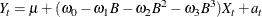This example models the effect of PRICE on SALES as a linear function of the current and three most recent values of PRICE. It is equivalent to a multiple linear regression of SALES on PRICE, LAG(PRICE), LAG2(PRICE), and LAG3(PRICE).

This is an example of a transfer function with one numerator factor. The numerator factors for a transfer function for an input series are like the MA part of the ARMA model for the noise series.

#### Denominator Factors

You can also use transfer functions with denominator factors. The denominator factors for a transfer function for an input series are like the AR part of the ARMA model for the noise series. Denominator factors introduce exponentially weighted, infinite distributed lags into the transfer function.

To specify transfer functions with denominator factors, place the denominator factors after a slash (/) in the INPUT= option. For example, the following statements estimate the PRICE effect as an infinite distributed lag model with exponentially declining weights:

   proc arima data=a;
identify var=sales crosscorr=price;
estimate input=( / (1) price );
run;


The transfer function specified by these statements is as follows: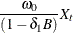This transfer function also can be written in the following equivalent form: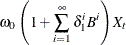This transfer function can be used with intervention inputs. When it is used with a pulse function input, the result is an intervention effect that dies out gradually over time. When it is used with a step function input, the result is an intervention effect that increases gradually to a limiting value.

#### Rational Transfer Functions

By combining various numerator and denominator factors in the INPUT= option, you can specify rational transfer functions of any complexity. To specify an input with a general rational transfer function of the form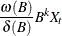use an INPUT= option in the ESTIMATE statement of the form

input=( k \$ (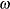-lags ) / (
-lags) x)